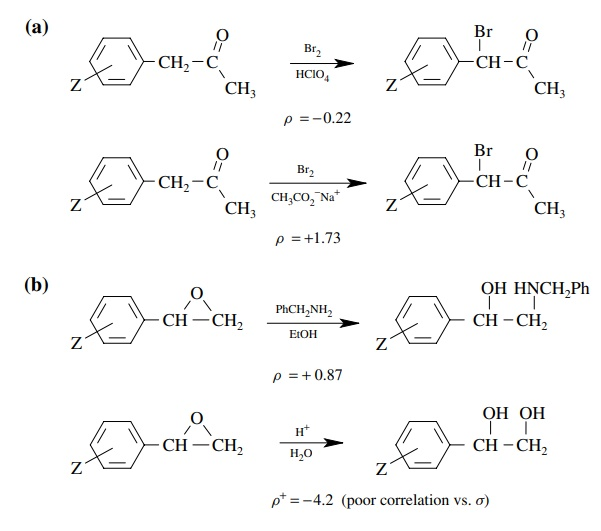# Solved Problems on Mechanisms of Organic Reactions

| Home | | Organic Chemistry |

## Chapter: Organic Chemistry : Mechanisms of Organic Reactions

Questions and Answers, Solved Problems on Mechanisms of Organic Reactions - Organic Chemistry : Mechanisms of Organic Reactions

PROBLEMS

5.1. The base-promoted elimination of quaternary ammonium ions (Hofmann elimination) has been proposed to proceed by an E2-like mechanism. Tell how each of the following observations supports this mechanistic classifi-cation. Be specific about exactly what each piece of information reveals.(a) The positive sign of ρ shows that an increase in electron density occurs during the transition state of the rate-determining step. The magnitude shows that there is a considerable electron density increase. Since the reagent responsible is the base ethoxide, it is pretty clear that significant benzylic proton removal by the base is occurring in the activated complex of the rate-determining step. Moreover proton removal is well advanced.

(b) This is a primary kinetic deuterium isotope effect, consistent with the notion that removal of the benzylic proton by base is taking place in the rate-determining step.

(c) This is also a primary kinetic isotope effect; only this is a carbon isotope effect. This means that cleavage of the carbon – nitrogen bond is occurring at the transition state of the rate-determining step. Since both proton removal and loss of the leaving group are occurring at the transition state, this then seems to be a concerted E2-type elimination.

(d) These results show that there is a distinct stereoelectronic requirement for this process — that the proton and the leaving group are antiperiplanar. This is typical of an E2-type base-promoted elimination. The major product is the result of the deuterium isotope effect, which results in faster removal of the proton than the deuterium.

5.2. Explain the mechanistic significance of the ρ values for the elimination of 1 under the two different sets of conditions.The positive value of ρ is indicative of increasing electron density on the benzylic carbon at the transition state of the rate-determining step. This is consistent with a mechanism in which the methoxide is acting as a base to remove the benzylic proton in the rate-determining step. The small value suggests that proton removal is not far advanced.

The second case with the negative value of ρ indicates that in this system a decrease in electron density occurs at the transition state. Ionization of the chloride leaving group to give a carbocation is consistent with this ρ value. The modest magnitude is consistent with the fact that charge formation occurs at the β position relative to the ring so the effect of substituents on charge formation is small. Also the correlation with σ is consistent since there is no direct resonance interaction of the developing charge with the aromatic ring due to the insulating methylene group.5.3. Propose a mechanism for the following reaction given that kH/ kD = 6.1 and ρ = 2.02 (there is a better correlation with σ substituent constants than with σ values).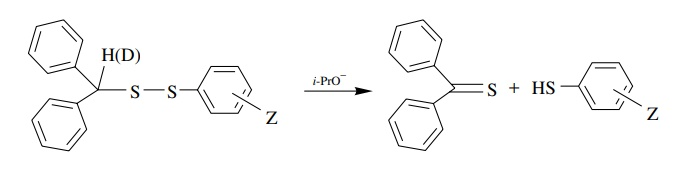The positive sign, magnitude, and correlation with σ indicate that there is a significant increase in electron density on an atom in conjugation with the aromatic ring. That would be the sulfur atom attached to the ring that is substituted. The primary kinetic deuterium isotope effect shows that cleavage of the C–H bond occurs in the rate-determining step. The best mechanism is a concerted, base-promoted elimination

5.4. Tell how you could use isotopes to distinguish between the following two mechanisms:Since the lower pathway has proton removal as an integral part of the mechanism, you could use isotopic substitution in two ways. First make the deuterated com-pound D. The kinetic deuterium isotope effect should be primary for the lower path but very near 1 for the upper path. Moreover, if you looked at the product, there should be no deuterium left in the product if the lower path is followed, whereas deuterium should be retained in the product if the addition elimination path is followed.

5.5. The following transformations occur by different mechanisms. Show them and predict what a Hammett study would show for each of them.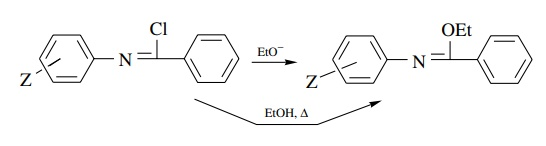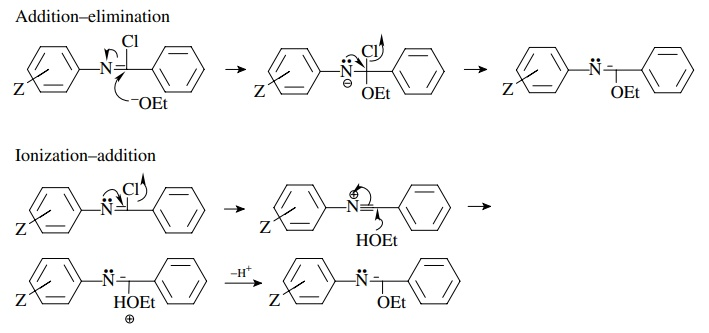In the first case the ρ value will be positive because of the increase in electron density on the nitrogen next to the aromatic ring and may correlate better with σ than with σ because of conjugation with the ring. In the second case, ionization would lead to a decrease in electron density on the nitrogen attached to the ring and would thus give a negative r value.

5.6. The base hydrolysis of a series of substituted phenoxy esters gave a much better correlation when the rate constants were plotted against σZ than against σZ. Give the mechanistic significance of this behavior.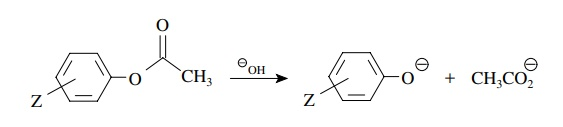The better correlation with σ indicates that increasing electron density in con-jugation with the aromatic ring is involved in the rate-determining step. For the two mechanistic steps of the hydrolysis, both should have positive ρ values but only the second has charge development in conjugation with the ring. Thus for this hydrolysis breakdown of the tetrahedral intermediate is rate determining.

5.7. Based on the better correlation of rates versus σZ+ and ρ+ = −1.03, give a likely mechanism for the following substitution: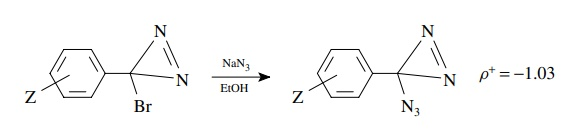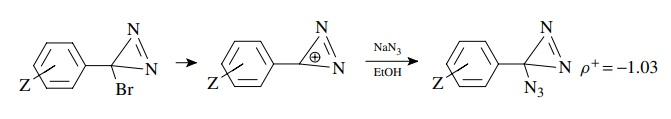The correlation with σ + and the negative slope indicate charge deficiency formed in conjugation with the ring. This in turn suggests an ionization mechanism in which ionization of bromide gives a cation. This cation might be particularly favored because it is an aromatic cation (Huckel) and would also explain the relatively low ρ+ value. The ρ + is low because the product cation is stabilized; thus the transition state is early with little charge development.

5.8. When 17α-methyl-5α-androstan-3β,17β-diol and its deuterated analog were tested, it was found that the protio compound is three times more active than the deutero analog. It is also known that the diol itself is not biologically active. What is the likely metabolically active material? Explain.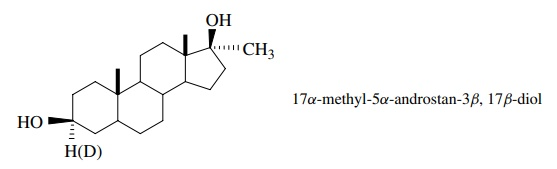The difference in activity for the protio and deutero compounds is likely a kinetic isotope effect of the transformation into the active compound. Since that involves removal of the methine proton, a reasonable deduction is that the ketone is the active species.

5.9. The oxidation of substituted benzaldehydes to substituted benzoic acids by pyridinium fluorochromate (PFC) has been studied, and it has been found that the reaction is first order with respect to pyridinium fluorochromate but is of complicated order with respect to the aldehyde. The following scenario was proposed to account for this behavior: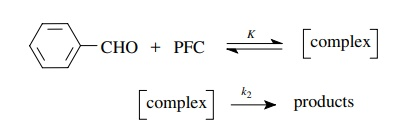Using the steady-state approximation, derive the rate law for this mecha-nistic scenario.

It was further found using α-deutero benzaldehyde that kH/ kD = 5.33 and using substituted benzaldehydes that ρ+ = −2.2:Use these data to delineate the nature of the rate-determining step and propose a plausible mechanism for the reaction. Explain how you used the data to arrive at the mechanism.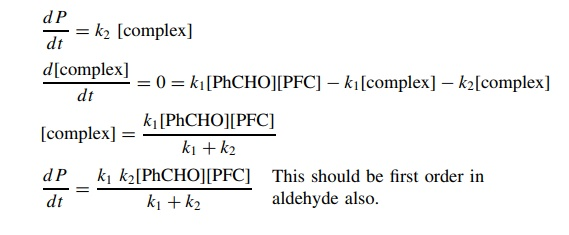The kinetic deuterium isotope effect indicates that C–H bond cleavage is part of the rate-determining step and the negative ρ value shows that there is electron defi-ciency being produced on the benzylic carbon in the activated complex. Therefore5.10. The rate of reaction of substituted aromatic chlorides with methoxide to produce substituted anisoles is found to give a linear correlation with σ but not with σZ. Explain in terms of a reaction mechanism.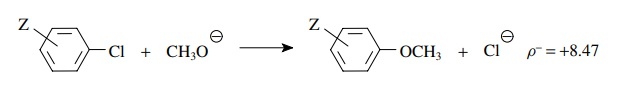The correlation with σ , the positive slope, and the relatively large magnitude of ρ means that a large charge density is built up on the ring in the activated complex of the rate-determining step. Addition of methoxide to give the Meisenheimer complex could account for this.

5.11. Derive the rate law that would describe the rate of product formation for the following reaction assuming that the cationic intermediate is a steady-state intermediate: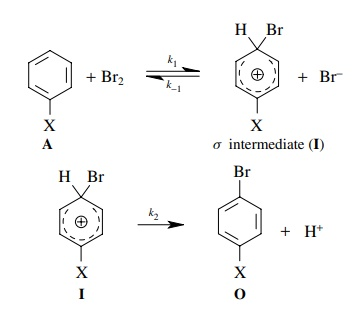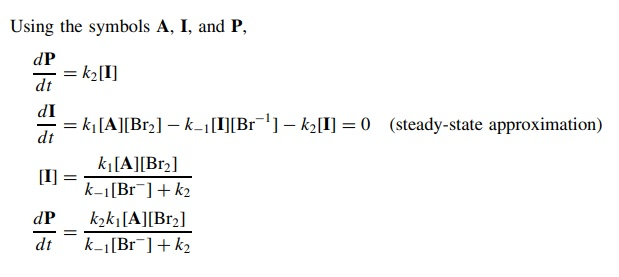5.12. Derive the rate law that would describe the rate of product formation for the following reaction where no assumptions are made as to the relative magnitudes of k1, k1, or k2:5.13. Benzaldehyde cyanohydrin formation shown below may involve rate-determining attack by either H+ or CN. A ρ value of +2.3 was found for the rate of formation of cyanohydrins from a series of substituted benzaldehydes. Which step is rate determining? Based on the fact that cyanohydrin formation does not occur in basic solution, write a complete mechanism for the process.Mechanism could beIf the protonation were rate determining, then a decrease in electron density at the carbonyl carbon occurs. The ρ value should be negative. If the second step is rate determining, then nucleophilic addition should result in an increase in electron density at the carbonyl group. the ρ value should be positive. The data show that the second step is probably rate determining. Moreover the lack of reaction under basic conditions means that the carbonyl group must be activated by protonation for the cyanide to add. Thus the protonation step is probably an equilibrium step.

5.14. For the following reactions tell which should have kH/ kD values greater than 1.5 for isotopic substitution at the starred hydrogens:(a) Since a base is used in the reaction and the base apparently removes the α proton, there should be a primary kinetic deuterium isotope effect of >1.5. For this to be true, proton removal must occur in the rate-determining step.

(b) In this case the base removes a proton from the molecule but the proton that is removed is not the one for which isotopic substitution has been done. Thus there should be only secondary isotope effects for this reaction of <1.5 (actually about 1.1).

(c) In this reaction a proton is lost from the molecule in order to form the product and that proton is one for which isotopic substitution is indicated. However, since there is no good base present to cause its removal, it is likely that the rate-determining step is ionization of the leaving group to give a carbocation. The proton is lost from this cation in a subsequent step. Since proton loss occurs after the rate-determining step, no primary kinetic deuterium isotope effect will be seen. Thus the isotope effect will be <1.5. Actually there is a secondary kinetic deuterium isotope effect of about 1.08.

5.15. For the solvolyses of A and B, rates of ionization were found to correlate best with ρ+ and gave the ρ+ values shown.

(a) How do the transition states for the solvolyses of A and B vary?

(b) How do you know this and why?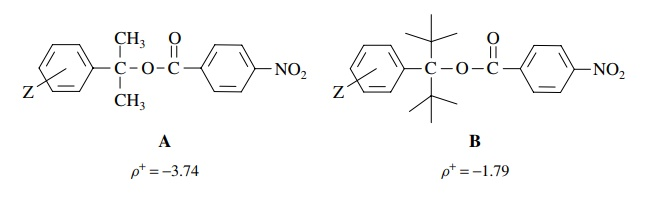(a) Since both correlate with σ + , there is a development of positive charge on the benzylic carbon which can be delocalized into the aromatic ring. The lower ρ value for B means that less electron deficiency is produced on the benzylic carbon of B than A.

(b) Since both of these tertiary, benzylic substrates solvolyze by ionization as the rate-determining step, the difference in the transition state parameters suggests that the transition state for the tert-butyl case B is much earlier. One reason could be that the product ion from B is more stable than the product ion from A, which would result in an earlier transition state by the Hammond postulate. This is not a likely explanation since both are tertiary benzylic carbocations as mentioned earlier. The difference in ρ values is probably related to steric factors. Since the bulky t -butyl groups tend to flatten out the normal tetrahedral geometry, B is strained relative to A and thus of higher energy. (This effect is actually termed B strain.) Thus the products are of similar energy but the reactants are not. The Hammond postulate predicts that the reactant of higher energy, B, will have an earlier transition state, as is seen.

5.16. Draw mechanisms for the following transformations using curved-arrow notation based on the data you are given. There may be more than one step.